# ggplot2: Text Annotations

### Introduction

This is the sixth post in the series Elegant Data Visualization with ggplot2. In the previous post, we learnt to modify the axis and plot labels. In this post, we will learn to add text to the plots.

• modify color
• modify size
• modify fontface
• modify angle

### Libraries, Code & Data

We will use the following libraries in this post:

All the data sets used in this post can be found here and code can be downloaded from here.

#### Annotate

We will use the `annotate()` function to add custom text to the plots. You can use the `annotate()` function to add rectangles/segments/pointrange as well but our focus will be on adding text. Let us start with a simple scatter plot.

`annotate()` takes the following arguments:

• `geom` : specify text
• `x` : x axis location
• `y` : y axis location
• `label` : custom text
• `color` : color of text
• `size` : size of text
• `fontface` : fontface of text
• `angle` : angle of text

Let us begin by adding text to a scatter plot. We will use the mtcars data set and continue to examine the relationship between displacement and miles per gallon. To add the text, we have to indicate that we are using `annotate()` for adding text, and we do this by ensuring that the first input is the word `'text'`. Now, ggplot2 knows that it should add a text to the plot but it still needs other information such as:

• where should the text appear on the plot i.e. location of the text
• and the text itself

We will provide the location by specifying points on the X and Y axis which are also the second and third inputs to `annotate()` and the final input is the text itself, which in our example is `'Sample Text'`.

``````ggplot(mtcars) +
geom_point(aes(disp, mpg)) +
annotate('text', x = 200, y = 30, label = 'Sample Text')``````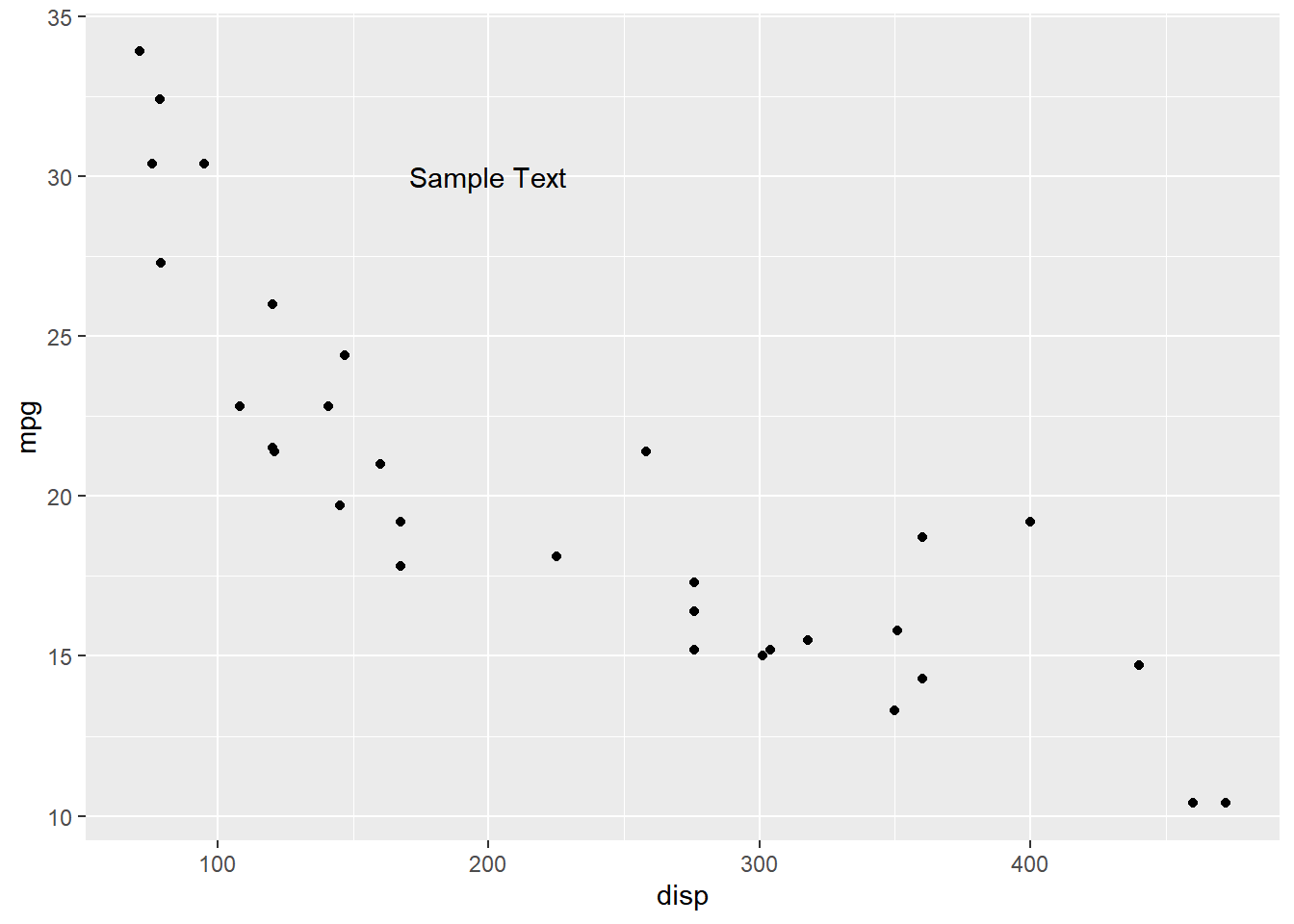#### Color

Now that we know how to add text, let us look at modifying the appearance of the text. To change the color, use the `color` argument. In the below example, we modify the color to `'red'`.

``````ggplot(mtcars) +
geom_point(aes(disp, mpg)) +
annotate('text', x = 200, y = 30, label = 'Sample Text', color = 'red')``````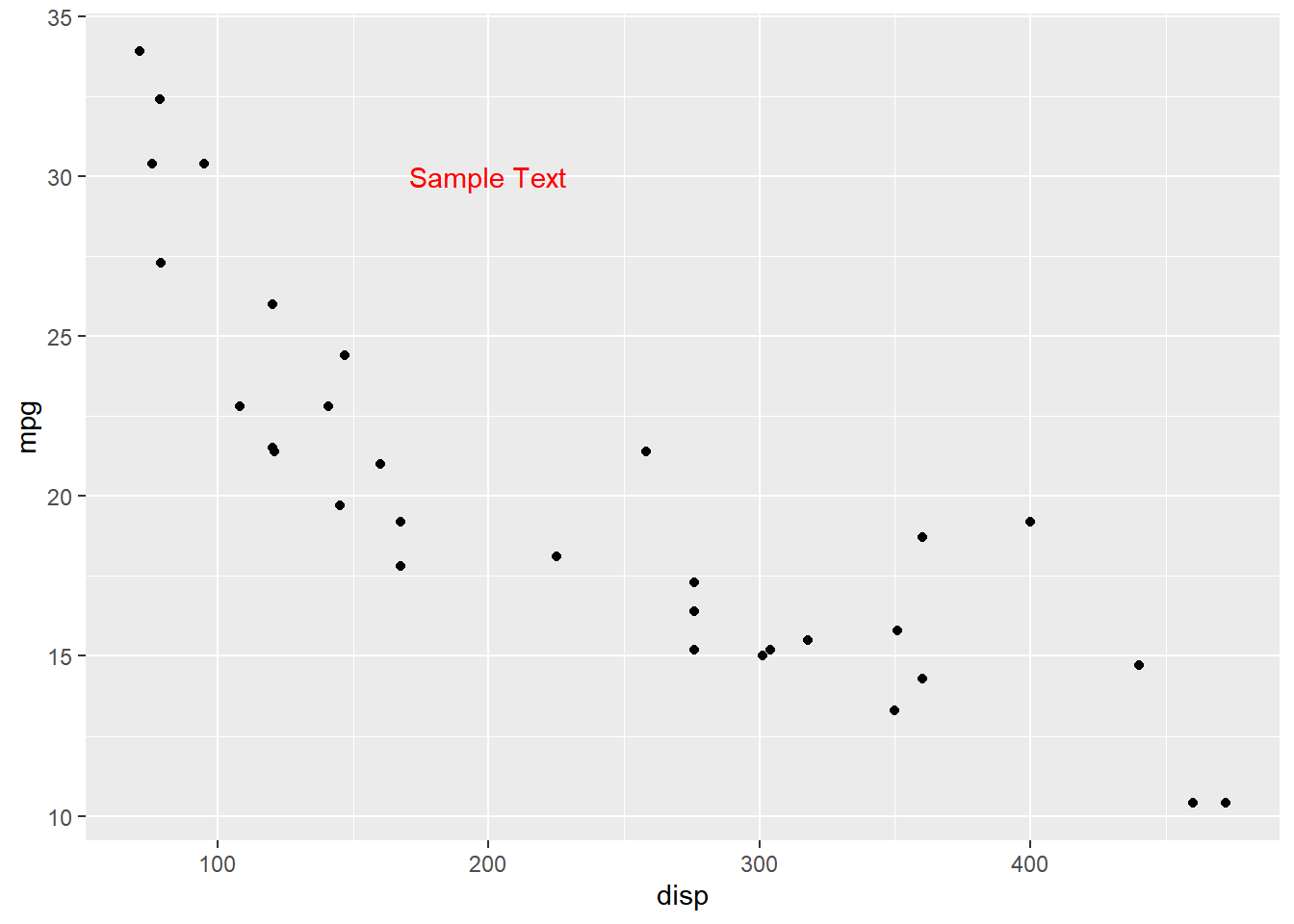#### Size

The `size` argument can be used to modify the size of the text.

``````ggplot(mtcars) +
geom_point(aes(disp, mpg)) +
annotate('text', x = 200, y = 30, label = 'Sample Text', size = 6)``````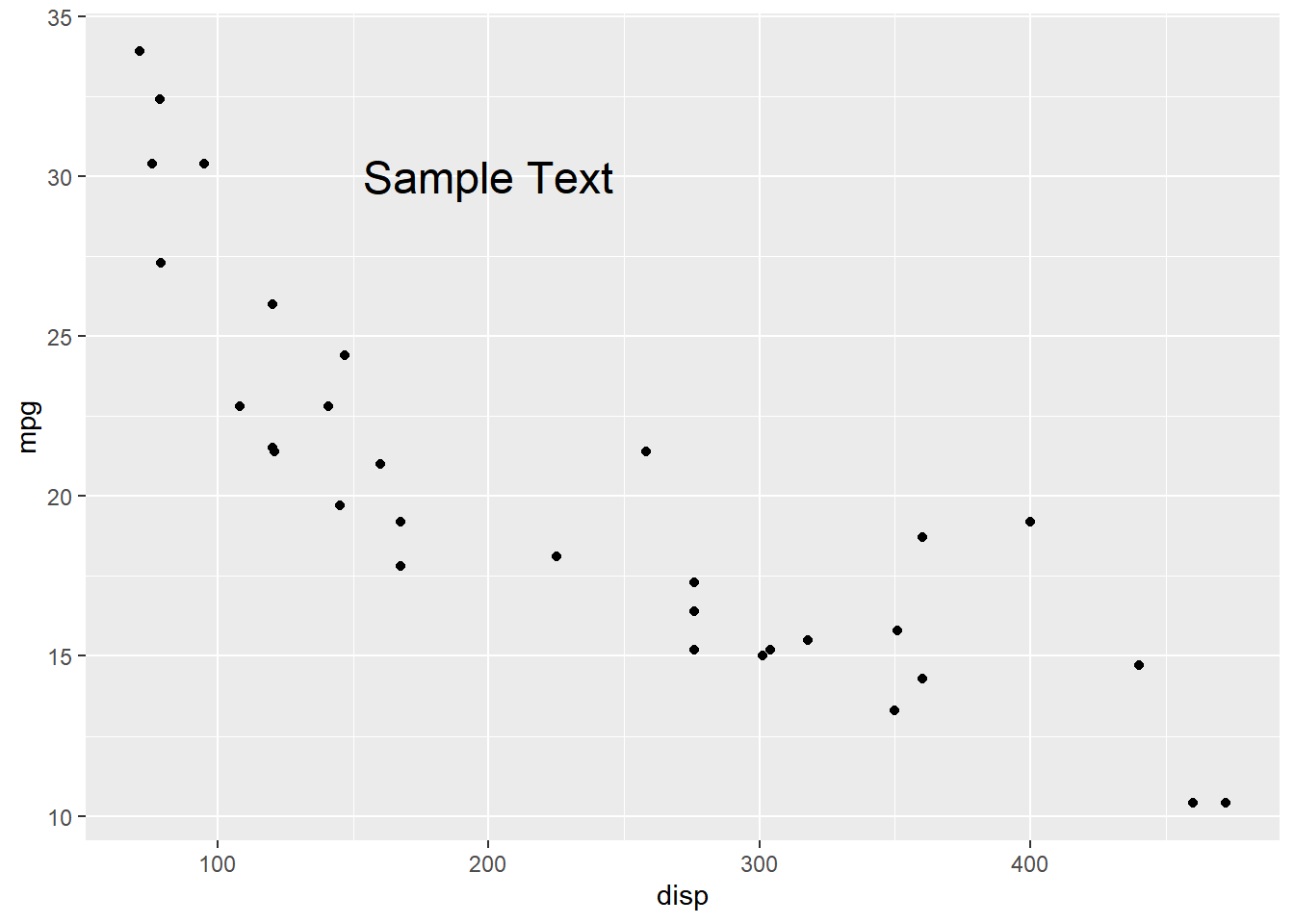#### Font

To choose a font of your liking, use the `font` argument and supply it a valid value.

``````ggplot(mtcars) +
geom_point(aes(disp, mpg)) +
annotate('text', x = 200, y = 30, label = 'Sample Text', fontface = 'bold')``````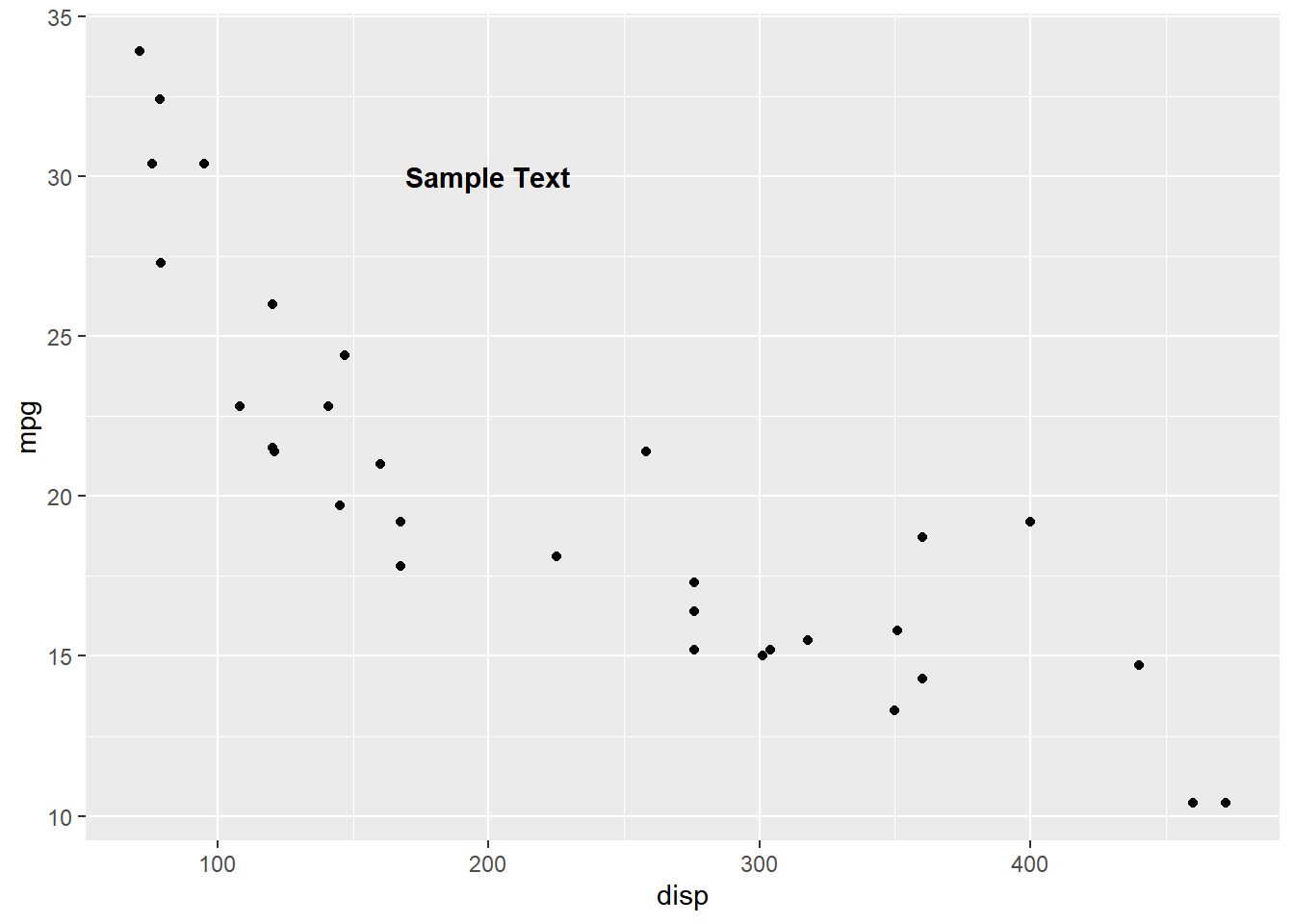#### Angle

The angle of the text can also be modified using the `angle` argument. In the below example, we modify the angle of the text to `25`.

``````ggplot(mtcars) +
geom_point(aes(disp, mpg)) +
annotate('text', x = 200, y = 30, label = 'Sample Text', angle = 25)``````#### Putting it all together..

``````ggplot(mtcars) +
geom_point(aes(disp, mpg)) +
annotate('text', x = 200, y = 30, label = 'Sample Text',
color = 'red', size = 6, fontface = 'bold', angle = 25)``````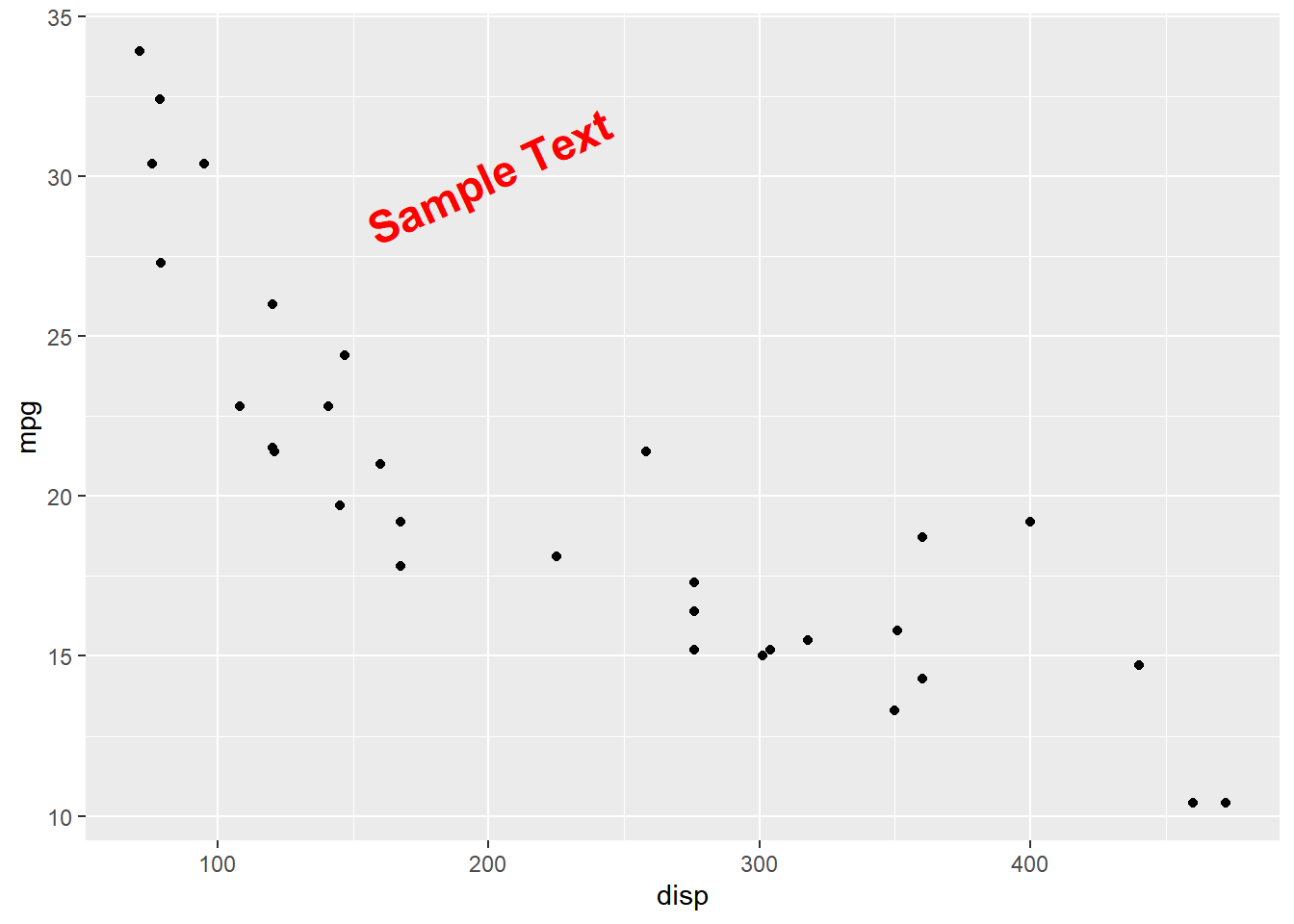#### Summary

In this post, we learnt to: